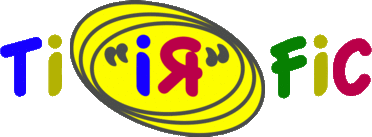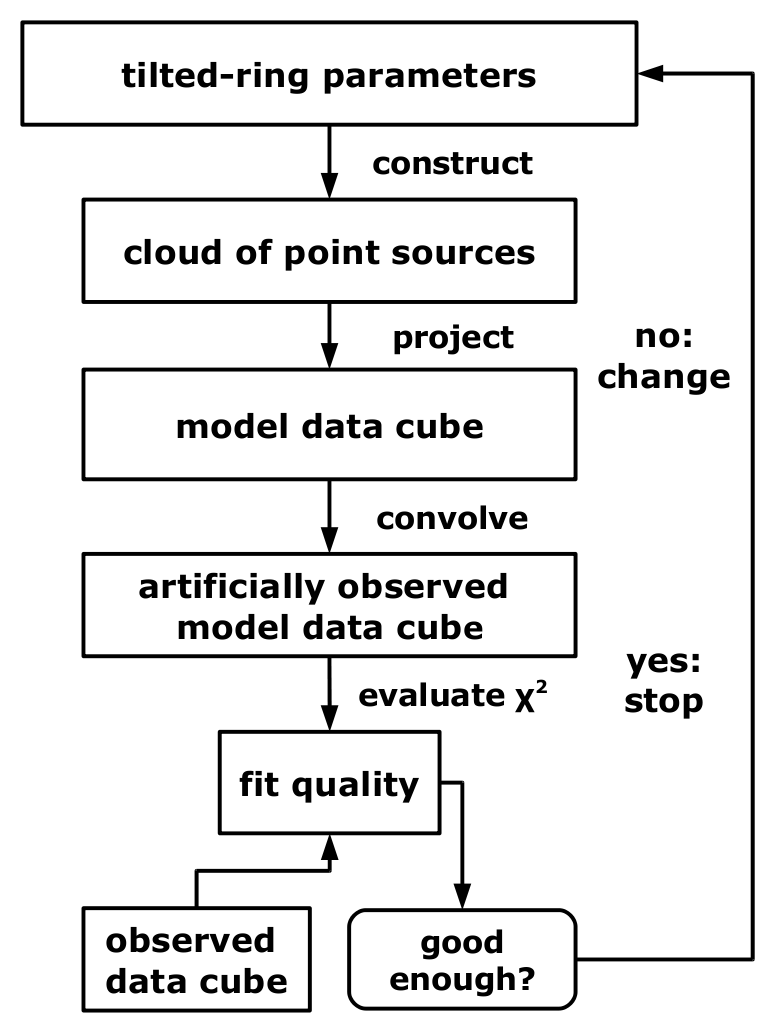Model FittingFig. 1: TiRiFiC model construction and fitting
The fitting strategies implemented in TiRiFiC are introduced in this section.

The flow diagram (Fig. 1) to the right shows how TiRiFiC is expanded to enable the user not only to construct simulated observations, but also to automatically adjust model parameters to fit the simulated observation to an input observation.

The difficulty is to implement a minimising algorithm, which is able to converge quickly, dealing with a complex $\chi^2$-landscape with a generally high number of parameters, a limited available amount of model calculations (since, after all optimisation for speed, the construction of one model still takes significantly longer than a µs), and without analytic (partial) derivatives. So far, three of several tested fitting methods yielded acceptable results.

Fitting strategy

General fitting concept

The aim of the fitting process in TiRiFiC is to adjust a subset of the geometrical parameters with the goal to optimise a simulated observation such that the simulated observation optimally matches a real (input) observation. The measure adopted to numerically determine the model quality is the $\chi^2$, altered by defining boundary conditions (minimum and maximum values for a parameter, regularisation schemes). Currently, the TiRiFiC optimisation aims at the minimisation of the $\chi^2$.

As far as possible, the fitting routines in TiRiFiC are kept separate from the model generation. This means that the fitting parameters, passed to the fitting routine are normalised (with a normalisation factor provided by the user through the parameter MINDELTA) to then be varied as dimensionless quantities on a regular grid. The parameters visible to the fitting routine are not necessarily mapped to single geometrical parameters parameters, but the user has the possibility to automatically adjust single geometrical parameters at given radii via linear interpolation between fitted quantities, and to group geometrical parameters to be fitted at the same time, in which case only one representative fitting variable is visible to the fitting routine. In other words, such a fitting group appears to the minimising routine as one parameter and automatically adjusted parameters are not visible at all to the fitting routine. Grouping and interpolation is controled by the the parameters VARY, VARINDX, and INDINTY .

In the following sections, we discuss the $\chi^2$ evaluation including the implementation of boundary conditions altering the $\chi^2$, to then describe the implemented fitting algorithms. Finally, the fitting parameters are described in detail.

Goodness-of-fit evaluation: basic

As a measure of the quality of a fit, TiRiFiC calculates the $\chi^2$ and with that, in principle, the relative probability of two models. The chisquare calculation before taking into account boundary conditions is determined by the WEIGHT and the RMS parameters. The $\chi^2$ calculates with

\begin{equation} \label{eq_2.1} \begin{split} \chi^2 &= \sum_i \frac{(M_i-O_i)^2}{\sigma_i^2} \\ &= \sum_i \frac{(M_i-O_i)^2}{w_i} \end{split} \qquad, \end{equation}

where $k$ is an index over all pixels, $(M_i)$ is the model data cube and $(O_i)$ the observed data cube, $(\sigma_i)$ the (pixel-dependent) noise, and $(w_i)$ the corresponding weighting function. If no quantisation noise is assumed, then

\begin{equation} \label{eq_2.2} \begin{split} \sigma_i &= \sigma_\mathrm{rms} \\ &= \mathrm{RMS} \end{split} \qquad, \end{equation}